# Derangement

(diff) ← Older revision | Latest revision (diff) | Newer revision → (diff)

derangement

A permutation ofelements in which the elementcannot occupy the-th position,. The problem of calculating the numberof derangements is known as the "problème des rencontresproblème des rencontres" . The following formula holds: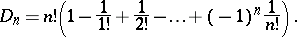Derangements are a special case of permutations satisfying a specific restriction on the position of the permuted elements. For example, the "problème des ménagesproblème des ménages" consists in calculating the number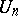of permutations conflicting with the two permutations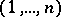and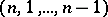. (Two permutations ofelements are called conflicting if the-th element occupies different positions in each of them for all). The numberis given by the formula:The numberof Latin squares (cf. Latin square) of size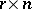for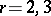can be calculated in terms of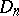andby the formulas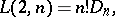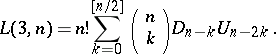How to Cite This Entry:
Derangement. Encyclopedia of Mathematics. URL: http://encyclopediaofmath.org/index.php?title=Derangement&oldid=18965
This article was adapted from an original article by V.M. Mikheev (originator), which appeared in Encyclopedia of Mathematics - ISBN 1402006098. See original article